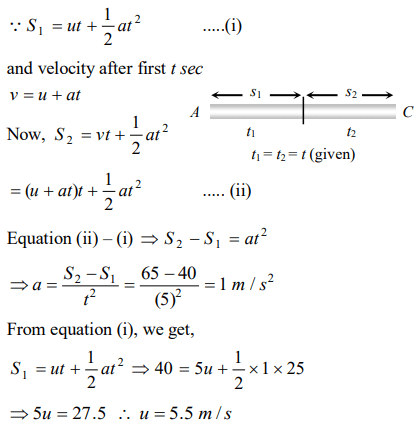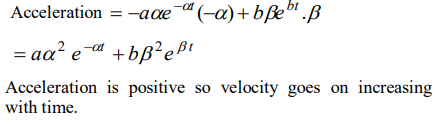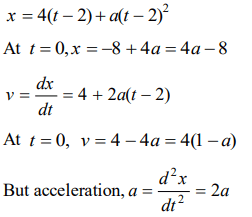## Motion in a Straight Line Questions and Answers Part-11

1. An object accelerates from rest to a velocity 27.5 m/s in 10 sec then find distance covered by object in next 10 sec
a) 550 m
b) 137.5 m
c) 412.5 m
d) 275 m

Explanation:2. If the velocity of a particle is given by $V=\left(180 -16 x\right)^{1/2}$    m/s, then its acceleration will be
a) Zero
b) $8 m\diagup s^{2}$
c) $-8 m\diagup s^{2}$
d) $4 m\diagup s^{2}$

Explanation:3. The displacement of a particle is proportional to the cube of time elapsed. How does the acceleration of the particle depends on time obtained
a) $a \propto t^{2}$
b) $a \propto 2t$
c) $a \propto t^{3}$
d) $a \propto t$

Explanation:4. Starting from rest, acceleration of a particle is $a =2\left(t-1\right)$  . The velocity of the particle at $t =5s$  is
a) 15 m/sec
b) 25 m/sec
c) 5 m/sec
d) None of these

Explanation:5. A body is moving with uniform acceleration describes 40 m in the first 5 sec and 65 m in next 5 sec. Its initial velocity will be
a) 4 m/s
b) 2.5 m/s
c) 5.5 m/s
d) 11 m/s

Explanation:6. Speed of two identical cars are u and 4u at a specific instant. The ratio of the respective distances in which the two cars are stopped from that instant is
a) 1 : 1
b) 1 : 4
c) 1 : 8
d) 1 : 16

Explanation:7. The displacement x of a particle varies with time $t,x =ae^{-\alpha t}+be^{\beta t}$    , where $a,b,\alpha$   and $\beta$ are positive constants. The velocity of the particle will
a) Go on decreasing with time
b) Be independent of $\alpha$ and $\beta$
c) Drop to zero when $\alpha =\beta$
d) Go on increasing with time

Explanation:8. A car, starting from rest, accelerates at the rate f through a distance S, then continues at constant speed for time t and then decelerates at the rate $\frac{f}{2}$ to come to rest. If the total distance traversed is 15 S, then
a) $S=\frac{1}{2}ft^{2}$
b) $S=\frac{1}{4}ft^{2}$
c) $S=\frac{1}{72}ft^{2}$
d) $S=\frac{1}{6}ft^{2}$

Explanation: Let car starts from point A from rest and moves up to point B with acceleration f9. A man is 45 m behind the bus when the bus start accelerating from rest with acceleration $2.5m\diagup s^{2}$. With what minimum velocity should the man start running to catch the bus ?
a) 12 m/s
b) 14 m/s
c) 15 m/s
d) 16 m/s10. A particle moves along x-axis as $x=4\left(t-2\right)+a\left(t-2\right)^{2}$